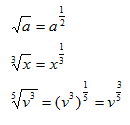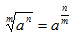Introduction

The last area of power functions to be addressed is the case of a fractional exponent. In order to express a radical expression without a radical sign, fractional (or rational) exponents are used. Some radical expressions and their equivalent rational exponent expressions are below:Fractional exponents are much easier to manipulate in solving equations than radicals, because all of the exponent rules apply to rational exponents. In general, roots can be written with fractional exponents in the following manner:

Roots as Fractional Exponents:The value on the radical becomes the denominator of the fractional exponent. The exponent on the value beneath the radical becomes the numerator of the fractional exponent.

Listen